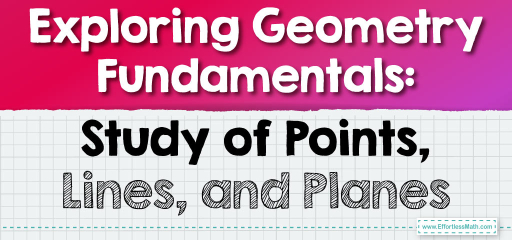# Exploring Geometry Fundamentals: Study of Points, Lines, and Planes

Geometry, a branch of mathematics that explores spatial relationships, begins with the most basic elements: points, lines, and planes. They are the fundamental constructs from which more advanced geometric shapes and concepts derive. As we unravel the intricacies of these primary elements, you'll find that understanding them is not just crucial for geometry but also offers insights into the spatial world around us. Let's embark on this exciting exploration!## Step-by-step Guide: Points, Lines, and Planes

Point:

Definition: A point in geometry represents a specific location. It does not have any size, width, or length.

Representation: It’s often denoted by a single capital letter, like $$A$$, $$B$$, or $$C$$.

Example: Consider a dot on a piece of paper; this can represent a point.

Line:
Definition: A line is a collection of points that extends infinitely in two directions. It has neither a starting point nor an ending point.

Representation: We usually represent a line with a lowercase letter or by naming two points on the line. For example, line $$l$$ or line $$AB$$ where $$A$$ and $$B$$ are points on the line.

Example: Think of a straight road that goes on forever in both directions.

Plane:
Definition: A plane is a flat surface that extends infinitely in all directions. Imagine it as a never-ending sheet.

Representation: Typically denoted by a single uppercase italic letter like plane $$M$$ or by naming three non-collinear points on the plane, for example, plane $$ABC$$.

Example: The surface of a calm lake, extending endlessly, can be visualized as a plane.

### Examples

Example 1:

If point $$A$$ lies on line $$m$$ and point $$B$$ also lies on line $$m$$, is it correct to represent the line as line $$AB$$?

Solution:

Yes, if both points $$A$$ and $$B$$ lie on line $$m$$, then we can represent the line as line $$AB$$.

Example 2:

If points $$X$$, $$Y$$, and $$Z$$ are on the same plane, and $$Y$$ and $$Z$$ are on Line $$n$$, what can we deduce?

Solution:

If $$X$$, $$Y$$, and $$Z$$ are on the same plane, say Plane $$P$$, then they all lie on the same flat surface. Since $$Y$$ and $$Z$$ are also on Line $$n$$, it implies that Line $$n$$ is entirely contained within Plane $$P$$. Point $$X$$ may or may not be on Line $$n$$, but it’s guaranteed to be on Plane $$P$$.

### Practice Questions:

1. If points $$A$$, $$B$$, and $$C$$ are on the same line, what term best describes their relationship?
2. Given Plane $$T$$ containing points $$D$$, $$E$$, and $$F$$, if Line $$k$$ passes through $$D$$ and $$E$$, where does Line $$k lie? 3. If point \(C$$ lies outside line $$DE$$, can we say that line $$DE$$ and point $$C$$ define a plane?
4. Represent a line passing through points $$F$$ and $$G$$.

1. Collinear.
2. Line $$k$$ lies entirely within Plane $$T$$.
3. Yes, any line and a point not on the line together define a unique plane.
4. The line can be represented as line $$FG$$.

### What people say about "Exploring Geometry Fundamentals: Study of Points, Lines, and Planes - Effortless Math: We Help Students Learn to LOVE Mathematics"?

No one replied yet.

X
30% OFF

Limited time only!

Save Over 30%

SAVE $5 It was$16.99 now it is \$11.99